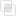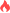python混合传递的基本原则2021-06-10 11:50:25790浏览 · 0收藏 · 0评论1、基本原则

（1）先按照参数的位置传递。

（2）再按照参数的关键字传递。

（3）最后按包裹的形式传递。

2、注意

3、实例

```func(1，2)  # 按位置传递方式将1、2赋值给a、b，c采用默认值0
a=1 b=2 c=0 args = () kw = {}
func(1, 2, c=3)  # 按位置传递方式将1、2赋值给a、b，将3赋值给c
a=1 b=2 c=3 args = () kw = {}
func(1, 2, 3, 'a', 'b')
a=1 b=2 c=3 args = ('a', 'b') kw = {}
func(1, 2, 3, 'a', 'b', x=99)
a=1 b=2 c=3 args = ('a', 'b') kw = {'x': 99}```python中Harris角点检测python字典键的两个特性python缺失值的解决方法python中Box-Cox变换是什么python中fail函数如何使用python中yaml文件的使用规则python中yaml的三种数据结构python中cookie和session的区别python HTTP和HTTPS的不同python有哪些常见的接口测试工具python函数中参数位置如何传递python关键字如何传递参数python异常中else的使用890

python捕获多个异常的规则1181

python ReLU函数是什么1235